Скачать презентацию How many tens and hundreds I am learning

1895fcdb35dd20d997674dda356ea922.ppt

• Количество слайдов: 5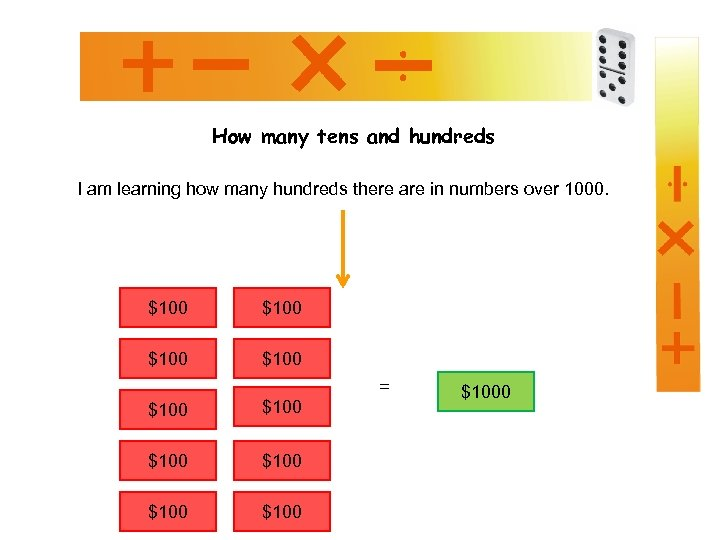How many tens and hundreds I am learning how many hundreds there are in numbers over 1000. \$100 \$100 \$100 = \$1000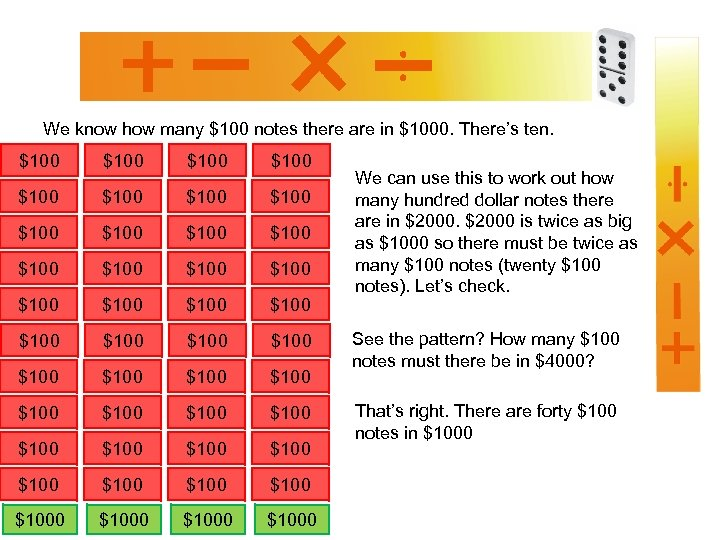We know how many \$100 notes there are in \$1000. There’s ten. \$100 \$100 \$100 \$100 \$100 \$100 \$100 \$100 \$100 \$100 \$1000 We can use this to work out how many hundred dollar notes there are in \$2000 is twice as big as \$1000 so there must be twice as many \$100 notes (twenty \$100 notes). Let’s check. See the pattern? How many \$100 notes must there be in \$4000? That’s right. There are forty \$100 notes in \$1000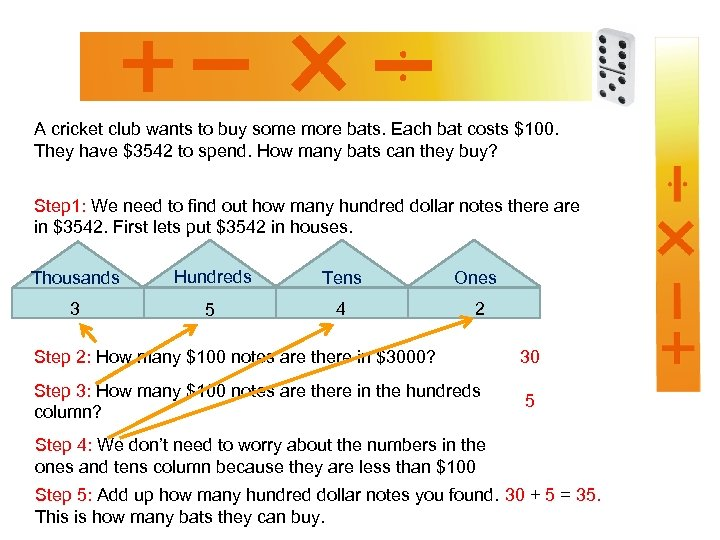A cricket club wants to buy some more bats. Each bat costs \$100. They have \$3542 to spend. How many bats can they buy? Step 1: We need to find out how many hundred dollar notes there are in \$3542. First lets put \$3542 in houses. Thousands Hundreds Tens Ones 3 5 4 2 Step 2: How many \$100 notes are there in \$3000? 30 Step 3: How many \$100 notes are there in the hundreds column? 5 Step 4: We don’t need to worry about the numbers in the ones and tens column because they are less than \$100 Step 5: Add up how many hundred dollar notes you found. 30 + 5 = 35. This is how many bats they can buy.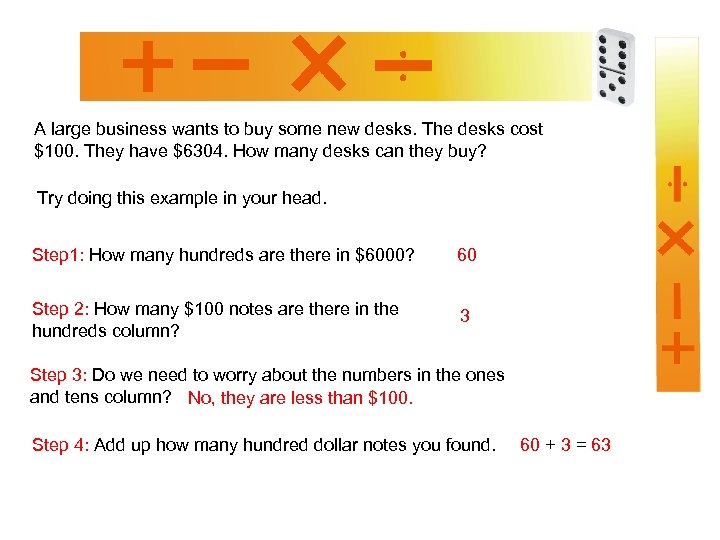A large business wants to buy some new desks. The desks cost \$100. They have \$6304. How many desks can they buy? Try doing this example in your head. Step 1: How many hundreds are there in \$6000? 60 Step 2: How many \$100 notes are there in the hundreds column? 3 Step 3: Do we need to worry about the numbers in the ones and tens column? No, they are less than \$100. Step 4: Add up how many hundred dollar notes you found. 60 + 3 = 63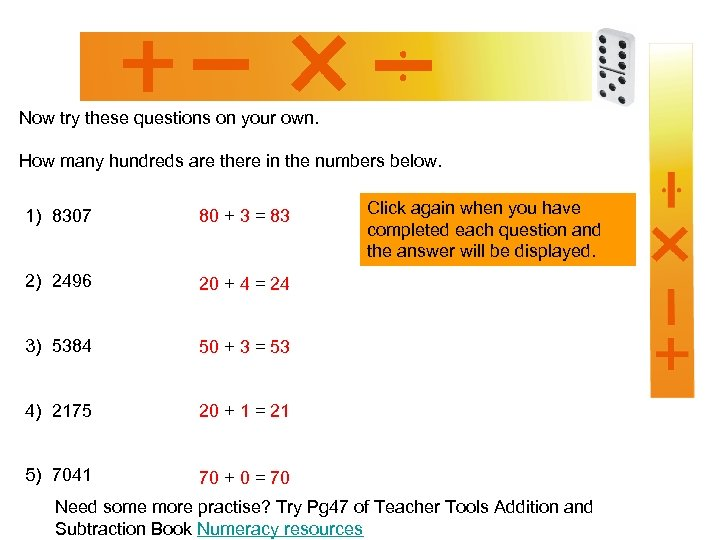Now try these questions on your own. How many hundreds are there in the numbers below. 1) 8307 80 + 3 = 83 2) 2496 20 + 4 = 24 3) 5384 50 + 3 = 53 4) 2175 20 + 1 = 21 5) 7041 Click again when you have completed each question and the answer will be displayed. 70 + 0 = 70 Need some more practise? Try Pg 47 of Teacher Tools Addition and Subtraction Book Numeracy resources